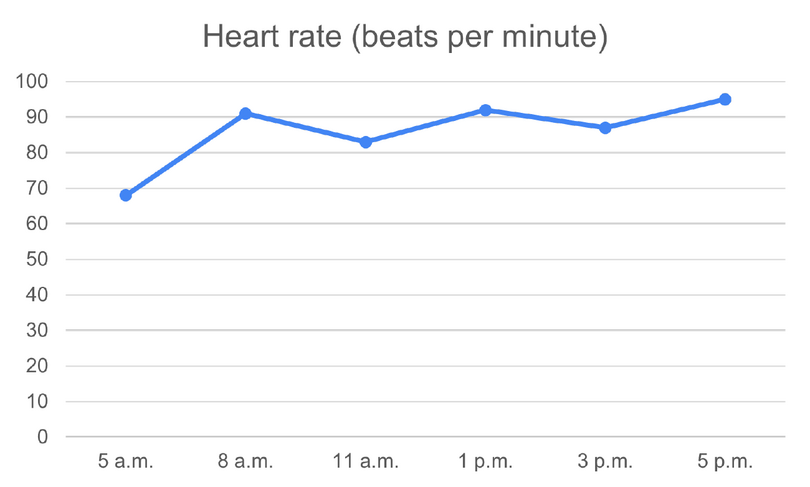Home Practice
For learners and parents For teachers and schools
Textbooks
Full catalogue
Pricing SupportLog in

We think you are located in United States. Is this correct?

# Broken-line graphs

## 19.5 Broken-line graphs

Broken-line graphs are data graphs that represent data points joined by a line. It is important to note that broken-line graphs are not the same as straight-line graphs.

Example of a broken-line graph Example of a straight-line graph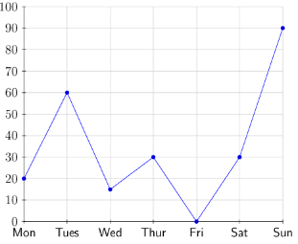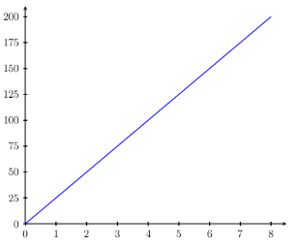Broken-line graphs are used to represent data that change continuously over time, such as the average daily temperature for a week, or a person’s heart-rate for a day (i.e. continuous data). Each data value is represented by a point on the graph, and the points are joined by straight line segments to make a broken-line graph.

Broken-line graphs are useful for identifying trends and patterns in data sets.

## Worked Example 19.6: Drawing a broken-line graph from a table

A group of friends went on a $$10$$-hour hike. They recorded the temperature at certain times during the hike. Represent the given information as a broken-line graph.

Time (hours) Temperature ($$^{\circ}\text{C}$$)
$$0$$ $$29$$
$$2$$ $$28$$
$$5$$ $$27$$
$$7$$ $$24$$
$$10$$ $$26$$

### Identify labels for the horizontal and vertical axes.

The table shows the time and the corresponding temperature reading during the hike. The time (in hours) will be on the horizontal axis and the temperature (in degrees Celsius) will be on the vertical axis. Notice that the change in temperature depends on time.

### Determine a suitable scale for the vertical axis.

First, we need to determine the range of temperature values. The lowest value is $$24 ^{\circ}\text{C}$$ and the highest value is $$29 ^{\circ}\text{C}$$. So for the vertical axis we can use a scale from $$0 ^{\circ}\text{C}$$ to $$32 ^{\circ}\text{C}$$ with intervals of $$2 ^{\circ}\text{C}$$.

### Draw the broken-line graph.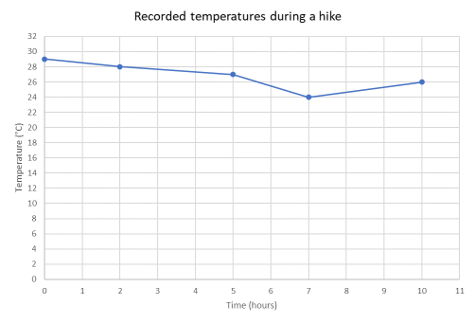# Test yourself now

High marks in science are the key to your success and future plans. Test yourself and learn more on Siyavula Practice.

Exercise 19.1

Use the broken-line graph to answer the following questions.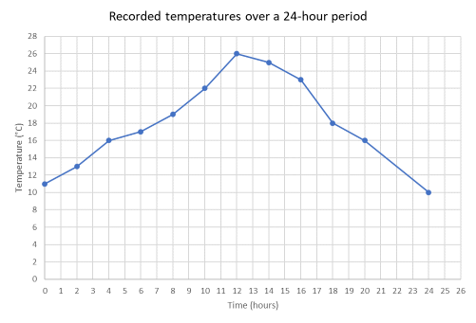1. What was the temperature after $$4$$ hours?
2. What was the minimum temperature during the $$24$$-hour period?
3. What was the temperature after $$10$$ hours?
4. What was the maximum temperature during the $$24$$-hour period?
5. At what time(s) was the temperature $$18 \text{ °C}$$?
1. $16 \text{ °C}$
2. $10 \text{ °C}$
3. $22 \text{ °C}$
4. $26 \text{ °C}$
5. At $$7$$ hours and $$18$$ hours

Represent the data given below as a broken-line graph.

Time ($$\text{h}$$) Temperature ($$^{\circ}\text{C}$$)
$$1$$ $$25$$
$$2$$ $$28$$
$$3$$ $$30$$
$$4$$ $$31$$
$$5$$ $$34$$
$$6$$ $$36$$
$$7$$ $$37$$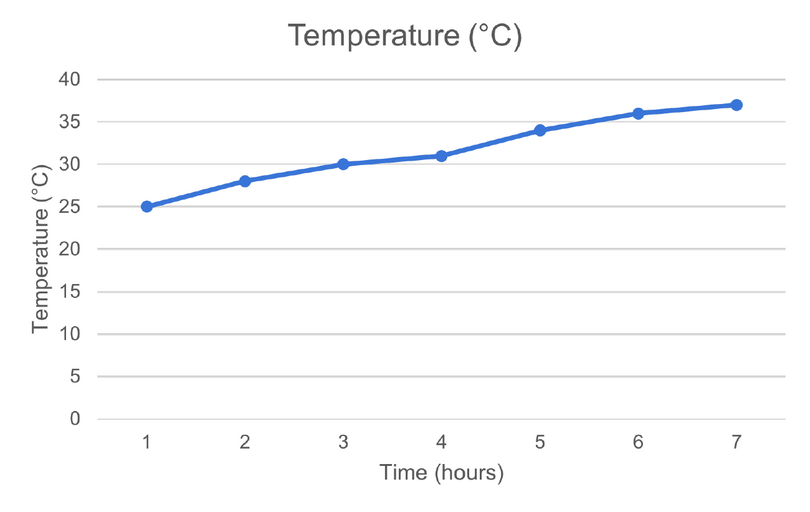Use the table of values to draw a broken-line graph.

Time Heart rate (beats per minute)
$$5$$ a.m. $$68$$
$$8$$ a.m. $$91$$
$$11$$ a.m. $$83$$
$$1$$ p.m. $$92$$
$$3$$ p.m. $$87$$
$$5$$ p.m. $$95$$